International
Tables for
Crystallography
Volume D
Physical properties of crystals
Edited by A. Authier

International Tables for Crystallography (2006). Vol. D, ch. 2.2, pp. 300-301

## Section 2.2.10. Density functional theory

K. Schwarza*

aInstitut für Materialchemie, Technische Universität Wien, Getreidemarkt 9/165-TC, A-1060 Vienna, Austria
Correspondence e-mail: kschwarz@theochem.tuwein.ac.at

### 2.2.10. Density functional theory

| top | pdf |

The most widely used scheme for calculating the electronic structure of solids is based on density functional theory (DFT). It is described in many excellent books, for example that by Dreizler & Gross (1990), which contains many useful definitions, explanations and references. Hohenberg & Kohn (1964) have shown that for determining the ground-state properties of a system all one needs to know is the electron density. This is a tremendous simplification considering the complicated wavefunction of a crystal with (in principle infinitely) many electrons. This means that the total energy of a system (a solid in the present case) is a functional of the density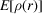, which is independent of the external potential provided by all nuclei. At first it was just proved that such a functional exists, but in order to make this fundamental theorem of practical use Kohn & Sham (1965) introduced orbitals and suggested the following procedure.

In the universal approach of DFT to the quantum-mechanical many-body problem, the interacting system is mapped in a unique manner onto an effective non-interacting system of quasi-electrons with the same total density. Therefore the electron density plays the key role in this formalism. The non-interacting particles of this auxiliary system move in an effective local one-particle potential, which consists of a mean-field (Hartree) part and an exchange–correlation part that, in principle, incorporates all correlation effects exactly. However, the functional form of this potential is not known and thus one needs to make approximations.

Magnetic systems (with collinear spin alignments) require a generalization, namely a different treatment for spin-up and spin-down electrons. In this generalized form the key quantities are the spin densities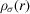, in terms of which the total energy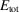is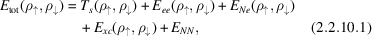with the electronic contributions, labelled conventionally as, respectively, the kinetic energy (of the non-interacting particles), the electron–electron repulsion, the nuclear–electron attraction and the exchange–correlation energies. The last term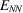is the repulsive Coulomb energy of the fixed nuclei. This expression is still exact but has the advantage that all terms but one can be calculated very accurately and are the dominating (large) quantities. The exception is the exchange–correlation energy, which is defined by (2.2.10.1)but must be approximated. The first important methods for this were the local density approximation (LDA) or its spin-polarized generalization, the local spin density approximation (LSDA). The latter comprises two assumptions:

 (i) Thatcan be written in terms of a local exchange–correlation energy density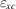times the total (spin-up plus spin-down) electron density as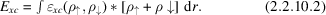(ii) The particular form chosen for. For a homogeneous electron gasis known from quantum Monte Carlo simulations, e.g. by Ceperley & Alder (1984). The LDA can be described in the following way. At each pointin space we know the electron density. If we locally replace the system by a homogeneous electron gas of the same density, then we know its exchange–correlation energy. By integrating over all space we can calculate.

The most effective way known to minimizeby means of the variational principle is to introduce (spin) orbitals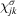constrained to construct the spin densities [see (2.2.10.7)below]. According to Kohn and Sham (KS), the variation ofgives the following effective one-particle Schrödinger equations, the so-called Kohn–Sham equations (Kohn & Sham, 1965) (written for an atom in Rydberg atomic units with the obvious generalization to solids):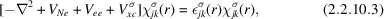with the external potential (the attractive interaction of the electrons by the nucleus) given by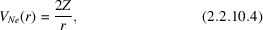the Coulomb potential (the electrostatic interaction between the electrons) given by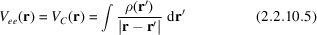and the exchange–correlation potential (due to quantum mechanics) given by the functional derivative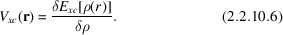In the KS scheme, the (spin) electron densities are obtained by summing over all occupied states, i.e. by filling the KS orbitals (with increasing energy) according to the Aufbau principle.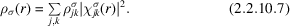Here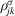are occupation numbers such that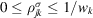, whereis the symmetry-required weight of point. These KS equations (2.2.10.3)must be solved self-consistently in an iterative process, since finding the KS orbitals requires the knowledge of the potentials, which themselves depend on the (spin) density and thus on the orbitals again. Note the similarity to (and difference from) the Hartree–Fock equation (2.2.9.1). This version of the DFT leads to a (spin) density that is close to the exact density provided that the DFT functional is sufficiently accurate.

In early applications, the local density approximation (LDA) was frequently used and several forms of functionals exist in the literature, for example by Hedin & Lundqvist (1971), von Barth & Hedin (1972), Gunnarsson & Lundqvist (1976), Vosko et al. (1980) or accurate fits of the Monte Carlo simulations of Ceperley & Alder (1984). The LDA has some shortcomings, mostly due to the tendency of overbinding, which causes, for example, too-small lattice constants. Recent progress has been made going beyond the LSDA by adding gradient terms or higher derivatives (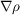and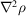) of the electron density to the exchange–correlation energy or its corresponding potential. In this context several physical constraints can be formulated, which an exact theory should obey. Most approximations, however, satisfy only part of them. For example, the exchange density (needed in the construction of these two quantities) should integrate toaccording to the Fermi exclusion principle (Fermi hole). Such considerations led to the generalized gradient approximation (GGA), which exists in various parameterizations, e.g. in the one by Perdew et al. (1996). This is an active field of research and thus new functionals are being developed and their accuracy tested in various applications.

The Coulomb potential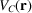in (2.2.10.5)is that of all N electrons. That is, any electron is also moving in its own field, which is physically unrealistic but may be mathematically convenient. Within the HF method (and related schemes) this self-interaction is cancelled exactly by an equivalent term in the exchange interaction [see (2.2.9.1)]. For the currently used approximate density functionals, the self-interaction cancellation is not complete and thus an error remains that may be significant, at least for states (e.g. 4f or 5f) for which the respective orbital is not delocalized. Note that delocalized states have a negligibly small self-interaction. This problem has led to the proposal of self-interaction corrections (SICs), which remove most of this error and have impacts on both the single-particle eigenvalues and the total energy (Parr et al., 1978).

The Hohenberg–Kohn theorems state that the total energy (of the ground state) is a functional of the density, but the introduction of the KS orbitals (describing quasi-electrons) are only a tool in arriving at this density and consequently the total energy. Rigorously, the Kohn–Sham orbitals are not electronic orbitals and the KS eigenvalues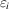(which correspond to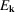in a solids) are not directly related to electronic excitation energies. From a formal (mathematical) point of view, theare just Lagrange multipliers without a physical meaning.

Nevertheless, it is often a good approximation (and common practice) to partly ignore these formal inconsistencies and use the orbitals and their energies in discussing electronic properties. The gross features of the eigenvalue sequence depend only to a smaller extent on the details of the potential, whether it is orbital-based as in the HF method or density-based as in DFT. In this sense, the eigenvalues are mainly determined by orthogonality conditions and by the strong nuclear potential, common to DFT and the HF method.

In processes in which one removes (ionization) or adds (electron affinity) an electron, one compares the N electron system with one with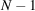or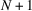electrons. Here another conceptual difference occurs between the HF method and DFT. In the HF method one may use Koopmans' theorem, which states that the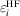agree with the ionization energies from state i assuming that the corresponding orbitals do not change in the ionization process. In DFT, thecan be interpreted according to Janak's theorem (Janak, 1978) as the partial derivative with respect to the occupation number,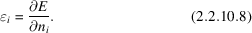Thus in the HF methodis the total energy difference for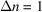, in contrast to DFT where a differential change in the occupation number defines, the proper quantity for describing metallic systems. It has been proven that for the exact density functional the eigenvalue of the highest occupied orbital is the first ionization potential (Perdew & Levy, 1983). Roughly, one can state that the further an orbital energy is away from the highest occupied state, the poorer becomes the approximation to useas excitation energy. For core energies the deviation can be significant, but one may use Slater's transition state (Slater, 1974), in which half an electron is removed from the corresponding orbital, and then use the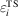to represent the ionization from that orbital.

Another excitation from the valence to the conduction band is given by the energy gap, separating the occupied from the unoccupied single-particle levels. It is well known that the gap is not given well by taking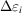as excitation energy. Current DFT methods significantly underestimate the gap (half the experimental value), whereas the HF method usually overestimates gaps (by a factor of about two). A trivial solution, applying the `scissor operator', is to shift the DFT bands to agree with the experimental gap. An improved but much more elaborate approach for obtaining electronic excitation energies within DFT is the GW method in which quasi-particle energies are calculated (Hybertsen & Louie, 1984; Godby et al., 1986; Perdew, 1986). This scheme is based on calculating the dielectric matrix, which contains information on the response of the system to an external perturbation, such as the excitation of an electron.

In some cases, one can rely on the total energy of the states involved. The original Hohenberg–Kohn theorems (Hohenberg & Kohn, 1964) apply only to the ground state. The theorems may, however, be generalized to the energetically lowest state of any symmetry representation for which any property is a functional of the corresponding density. This allows (in cases where applicable) the calculation of excitation energies by taking total energy differences.

Many aspects of DFT from formalism to applications are discussed and many references are given in the book by Springborg (1997).

### References

Barth, U. von & Hedin, L. (1972). A local exchange-correlation potential for the spin-polarized case: I. J. Phys. C, 5, 1629–1642.
Ceperley, D. M. & Alder, B. J. (1984). Ground state of the electron gas by a stochastic method. Phys. Rev. Lett. 45, 566–572.
Dreizler, R. M. & Gross, E. K. U. (1990). Density functional theory. Berlin, Heidelberg, New York: Springer-Verlag.
Godby, R. W., Schlüter, M. & Sham, L. J. (1986). Accurate exchange-correlation potential for silicon and its discontinuity of addition of an electron. Phys. Rev. Lett. 56, 2415–2418.
Gunnarsson, O. & Lundqvist, B. I. (1976). Exchange and correlation in atoms, molecules, and solids by the spin-density-functional formation. Phys. Rev. B, 13, 4274–4298.
Hedin, L. & Lundqvist, B. I. (1971). Explicit local exchange-correlation potentials. J. Phys. C, 4, 2064–2083.
Hohenberg, P. & Kohn, W. (1964). Inhomogeneous electron gas. Phys. Rev. 136, B864–B871.
Hybertsen, M. S. & Louie, G. (1984). Non-local density functional theory for the electronic and structural properties of semiconductors. Solid State Commun. 51, 451–454.
Janak, J. F. (1978). Proof that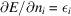in density-functional theory. Phys. Rev. B, 18, 7165–7168.
Kohn, W. & Sham, L. J. (1965). Self-consistent equations including exchange. Phys. Rev. 140, A1133–A1138.
Parr, R., Donnelly, R. A., Levy, M. & Palke, W. A. (1978). Electronegativity: the density functional viewpoint. J. Chem. Phys. 68, 3801–3807.
Perdew, J. P. (1986). Density functional theory and the band gap problem. Int. J. Quantum Chem. 19, 497–523.
Perdew, J. P., Burke, K. & Ernzerhof, M. (1996) Generalized gradient approximation made simple. Phys. Rev. Lett. 77, 3865–3868.
Perdew, J. P. & Levy, M. (1983). Physical content of the exact Kohn–Sham orbital energies: band gaps and derivative discontinuities. Phys. Rev. Lett. 51, 1884–1887.
Slater, J. C. (1974). The self-consistent field for molecules and solids. New York: McGraw-Hill.
Springborg, M. (1997). Density-functional methods in chemistry and material science. Chichester, New York, Weinheim, Brisbane, Singapore, Toronto: John Wiley and Sons Ltd.
Vosko, S. H., Wilk, L. & Nusair, M. (1980). Accurate spin-dependent electron liquid correlation energies for local spin density calculations. Can. J. Phys. 58, 1200–1211.